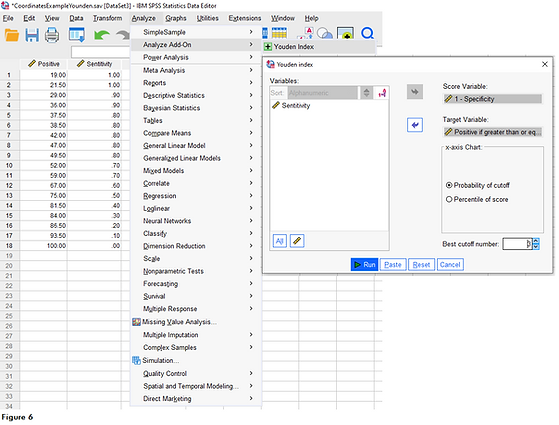top of page##### Youden Module of Statistics for Data Analysis

The new Youden Premium Add-On helps you determine the optimal cut-off of an ROC curve, a very useful technique that shows classification performance at different probability thresholds.

This technique is widely used in medicine, radiology, psychology, meteorology, veterinary medicine, and physics.

Reference to the ROC curve

The ROC (Receiver Operating Characteristic) curve is a graph that relates the sensitivity and the specificity of a diagnostic test to the change in a threshold value, also called the cut-off value.

The ROC curve analysis of a diagnostic test allows:

• To assess accuracy

• To determine the value of a more appropriate cut-off

• To compare the performance of two or more tests

To sample the importance of the Youden index, we will first recall some basic concepts that are essential to understand the topic.

For convenience, we state below some basic concepts:

• The goal of a test is to correctly classify a patient (e.g. presence or absence of a specific disease)

• Misclassified cases are called false positives and false negatives

• The sensitivity of a diagnostic test is the proportion of true positive cases that are correctly classified (e.g., in medicine it is the ability to correctly classify sick individuals).

• The specificity of a diagnostic test is the proportion of true negatives that are correctly classified (for example, in medicine it is the probability of correctly classifying healthy people).

# The ROC curve and diagnostics

The ROC curve is a very useful tool for summarizing in a single graph the performance of a diagnostic test as the cut-off value changes.

The graph of an ROC curve consists of:

• The sensitivity values, that is, the proportion of true positives of the test on the y-axis (y-axis)

• The specificity values, i.e., the proportion of false positives of the test on the x-axis (x-axis)

• within the graph each cut-off value is represented, for each of which the sensitivity value (on the y-axis) and specificity value (on the x-axis) can be read

• Connecting the various points gives a curve with a "staircase" pattern, the ROC curve.

The area under the ROC curve (AUC, short for "Area Under the Curve") is a measure of diagnostic accuracy. For simplicity, we could say that if a hypothetical new test discriminated sick people from healthy people perfectly, the area of the ROC curve would have value 1, that is, 100% accuracy. In the case where the new test did not discriminate sick from healthy at all, the ROC curve would have an area of 0.5 (or 50%), which would coincide with the area below the diagonal of the graph. In reality, a diagnostic test with an area under the curve ≥80% is considered adequate.

Very useful diagnostics is also the determination of the optimal cut-off, that is, the value that simultaneously maximizes the sensitivity and specificity of the test. This is the Youden index, which we see below with an example.

Richimo curva ROC
Curva ROc e diagnostiche

Example

Consider a hypothetical sample of 25 patients with terminal kidney disease. Two cardiac biomarkers (atrial natriuretic peptide, ANP, and brain natriuretic peptide, BNP) were measured for each patient, and the presence/absence of left ventricular hypertrophy was ascertained by echocardiography. ANP is mainly produced by the atrium, while BNP by the left ventricle, and there is evidence in the literature that these two biomarkers have good diagnostic power to identify left ventricular hypertrophy in dialysis patients (Figure 1).

EsempioTo draw the ROC curve graph, it is necessary to calculate the sensitivity, specificity and proportion of false positives (1-specificity) relative to a set of threshold values of ANP and BNP. For example, to calculate the coordinates of the ROC curve for ANP alone with Statistics for Data Analysis, one calls up the ROC Analyses dialog box from the Analyze/ Analyze ROC Menu (Figure 2).For brevity, figure 3 shows the coordinates of the ROC curve for ANP only.For example, an ANP cut-off of 59 pg/mL has a sensitivity of 70%, for identifying patients with left ventricular hypertrophy, and a false-positive rate of 20%. Reporting in the graph

all possible pairs of true positives and false positives, corresponding to each threshold value, the ROC curve is obtained (Figure 4).Figure 5 shows the AUC, which is 0.743 (i.e., 74%). This means that in a hypothetical experiment of randomly choosing in 100 different trials a pair of patients one of whom has left ventricular hypertrophy and one without, in 74% of cases ANP levels are higher in individuals with left ventricular hypertrophy than in those without this alteration.Indice Youden

Youden Index

Through the coordinates of the ROC curve, it is possible to identify the best cut-off, i.e., the test value that maximizes the difference between true positives and false positives, i.e., Youden's Test, available only in the Statistics for Data Analysis solution.

Once the coordinates of the ROC Curve have been reported on the dataset (Figure 7), it is possible to calculate the Youden Test thanks to the relevant Add-On, also being able to retrieve all the best results oneIn our case, the best cut-off is associated with a false-positive rate of 27 percent, as shown in the first row of the cutoff_score in Figure 7.This best cut-off corresponds to a value for ANP of 52 pg/mL, which is associated with a sensitivity of 70%. (Figure 8).In conclusion, in our example, this value derived from the Add-On of Youden's Test for ANP of 52 pg/mL is the one that maximizes the difference between true positives and false positives for the identification of left ventricular hypertrophy.

(The above example is only intended to show how to recall an ROC analysis and some of its diagnostics, including the Youden Index. Consider the invention data, with nothing to prove medically).

bottom of page